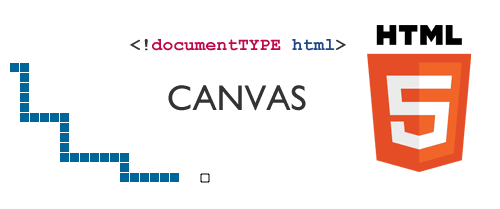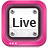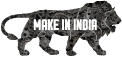HTML5 Snake Game
Wednesday, August 17, 2011

HTML5 Snake Game

Last some days I have been working with HTML5 canvas, sure it’s going to change the web future. I had implemented a snake game with HTML5 canvas + Javascript. Just take a look this live demo, it’s simple and light weight code you can use this script in 404 error pages and site down maintenance.Live Demo

DeveloperArun Kumar Shekar
Engineer
Chennai, INDIA

Code
This code contains three functions play_game(), rand_frog() and set_game_speed(). If you want to change the game theme, modify these five variable values such as level, rect_w, rect_h, inc_score and snake_color.
<!documentTYPE html>
<html lang="en">
<meta charset="utf-8" />
<title>Play Snake Game</title>
<style type="text/css">
body {text-align:center;}
canvas { border:5px dotted #ccc; }
h1 { font-size:50px; text-align: center; margin: 0; padding-bottom: 25px;}
</style>
<script type="text/javascript">
function play_game()
{
var level = 160; // Game level, by decreasing will speed up
var rect_w = 45; // Width
var rect_h = 30; // Height
var inc_score = 50; // Score
var snake_color = "#006699"; // Snake Color
var ctx; // Canvas attributes
var tn = []; // temp directions storage
var x_dir = [-1, 0, 1, 0]; // position adjusments
var y_dir = [0, -1, 0, 1]; // position adjusments
var queue = [];
var frog = 1; // defalut food
var map = [];
var MR = Math.random;
var X = 5 + (MR() * (rect_w - 10))|0; // Calculate positions
var Y = 5 + (MR() * (rect_h - 10))|0; // Calculate positions
var direction = MR() * 3 | 0;
var interval = 0;
var score = 0;
var sum = 0, easy = 0;
var i, dir;
// getting play area
var c = document.getElementById('playArea');
ctx = c.getContext('2d');
// Map positions
for (i = 0; i < rect_w; i++)
{
map[i] = [];
}
// random placement of snake food
function rand_frog()
{
var x, y;
do
{
x = MR() * rect_w|0;
y = MR() * rect_h|0;
}
while (map[x][y]);
map[x][y] = 1;
ctx.fillStyle = snake_color;
ctx.strokeRect(x * 10+1, y * 10+1, 8, 8);
}
// Default somewhere placement
rand_frog();
function set_game_speed()
{
if (easy)
{
X = (X+rect_w)%rect_w;
Y = (Y+rect_h)%rect_h;
}
--inc_score;
if (tn.length)
{
dir = tn.pop();
if ((dir % 2) !== (direction % 2))
{
direction = dir;
}
}
if ((easy || (0 <= X && 0 <= Y && X < rect_w && Y < rect_h)) && 2 !== map[X][Y])
{
if (1 === map[X][Y])
{
score+= Math.max(5, inc_score);
inc_score = 50;
rand_frog();
frog++;
}
//ctx.fillStyle("#ffffff");
ctx.fillRect(X * 10, Y * 10, 9, 9);
map[X][Y] = 2;
queue.unshift([X, Y]);
X+= x_dir[direction];
Y+= y_dir[direction];
if (frog < queue.length)
{
dir = queue.pop()
map[dir][dir] = 0;
ctx.clearRect(dir * 10, dir * 10, 10, 10);
}
}
else if (!tn.length)
{
var msg_score = document.getElementById("msg");
msg_score.innerHTML = "Thank you for playing game.<br /> Your Score : <b>"+score+"</b><br /><br /><input type='button' value='Play Again' onclick='window.location.reload();' />";
document.getElementById("playArea").style.display = 'none';
window.clearInterval(interval);
}
}
interval = window.setInterval(set_game_speed, level);
document.onkeydown = function(e) {
var code = e.keyCode - 37;
if (0 <= code && code < 4 && code !== tn)
{
tn.unshift(code);
}
else if (-5 == code)
{
if (interval)
{
window.clearInterval(interval);
interval = 0;
}
else
{
interval = window.setInterval(set_game_speed, 60);
}
}
else
{
dir = sum + code;
if (dir == 44||dir==94||dir==126||dir==171) {
sum+= code
} else if (dir === 218) easy = 1;
}
}
}
</script>
<h1>Play Snake Game</h1>
<div id="msg"></div>
<canvas id="playArea" width="450" height="300">Sorry your browser does not support HTML5</canvas>
</body>
</html>

1.When you pause the game, the speed of the snake is still increasing, so if you pause and come back you will have a ridiculous snake with the speed of the wind =)

2.3.Good one Arun

4.Awesome it nice

5.6.Would have been better if you had explain the things a little. Anyway great post. Waiting for some kind of tutorial stuff on this post.

7.8.9.Good post :D
I don't know if HTML5 can do this...

10.This is fun haha!

11.hihihi...for taking the time's...xixixixi

12.Awesome work... really!! I am alos web developer. n also want to do these kind of stuff... by learning jquery and html5 :)
gud luck Arun

13.nice..great job u have done..

14.It's really refreshing to see how jquery and HTML 5 work together. The possibilities are endless and would love to see more of such tutorials.

15.great tutorial, very nice job

16.nice. good script.

17.Indeed, its a javascript game not an html5 game dude...

1.Well, how do explain the canvas tag?

2.This is really amazing, Thanks a lot

3.18.19.awesome

20.A more complex version, based on the exact same technology can be found here -> http://www.helpfulsheep.com/snake/

21.22.Great Work!!!

23.loved the Game ..
a solution to the very fast return after a pause .. is that in line

interval = window.setInterval(set_game_speed, 60);

make it ..
interval = window.setInterval(set_game_speed, level);

and this will get you back to the old lever where you have been ;)

24.loved the Game ..
a solution to the very fast return after a pause .. is that in line

interval = window.setInterval(set_game_speed, 60);

make it ..
interval = window.setInterval(set_game_speed, level);

and this will get you back to the old lever where you have been ;)

25.you are really awesome..

26.Great stuff been trying to do something like this for ages.lol

27.how to calculate the directions can u explain in brief?

28.Thanks a lot

29.where do you change the color for the frogs?

30.how do you change the color of the food?

thanks

31.Thank you .... ; :D :D :D :D :D

32.this html cod das not be runinthe brwther ithink thiris asort of problem in this cod ples send other html cod ifyou have

33.THANKS FOR HTML CODE

34.hi THANKS FOR HTML CODE

35.